Next: References Up: TOMOGRAPHY AND OTHER APPLICATIONS Previous: Clash in philosophies

## An aside on theory-of-constraint equations

A theory exists for general constraints in quadratic form minimization. I have not found the theory to be useful in any application I have run into so far, but it should come in handy for writing erudite theoretical articles.

Constraint equations are an underdetermined set of equations, say(the number of components inexceeds that in), which must be solved exactly while some other set is solved in the least-squares sense, say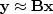.This is formalized as(15)
In my first book (FGDP: see page 113), I minimized QC by power series, letting, and hence.I minimized both Q(0) and Q(1) with respect to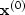and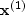.After a page of algebra, this approach leads to the system of equations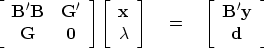(16)
wherehas been superseded by the variable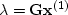,which has fewer components than,and wherehas simply been replaced by.The second of the two equations shows that the constraints are satisfied. But it is not obvious from equation (16) that (15) is minimized.

The great mathematician Lagrange apparently looked at the result, equation (16), and realized that he could arrive at it far more simply by extremalizing the following quadratic form: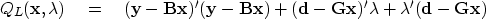(17)
We can quickly verify that Lagrange was correct by setting to zero the derivatives with respect to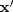and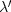.Naturally, everyone prefers to handle constraints by Lagrange's method. Unfortunately, Lagrange failed to pass on to the teachers of this world an intuitive reason why extremalizing (17) gives the same result as extremalizing (15). Lagrange's quadratic form is not even positive definite (that is, it cannot be written as something times its adjoint). In honor of Lagrange, the variableshave come to be known as Lagrange multipliers.Next: References Up: TOMOGRAPHY AND OTHER APPLICATIONS Previous: Clash in philosophies
Stanford Exploration Project
10/21/1998